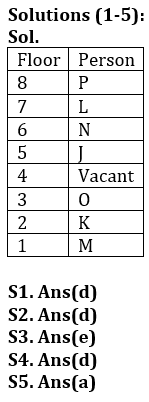Latest Banking jobs   »

# Reasoning Ability Quiz For Bank Foundation 2023-19th March

Directions (1-5): Study the information carefully and answer the questions given below.
Seven persons J, K, L, M, N, O and P live in eight floor building in such a way ground floor is no. 1 and top floor is no. 8 but not necessary in same order. One of the floors is vacant.
Three floors between L and O who lives at odd no. floor below floor no. 4. J lives just above vacant floor. Two persons live between J and M. L lives above O. J lives on odd no. floor. Vacant floor is not just above or just below L’s floor. K lives just above M floor. More than two floor between K and N. P lives above N.

Q1. Who among the following person live at 5TH floor?
(a) K
(b) M
(c) O
(d) J
(e) None of these

Q2. Who among the following lives just below the vacant floor?
(a) P
(b) K
(c) L
(d) O
(e) None of these

Q3. N lives on which of the following floor?
(a) 3rd
(b) 4th
(c) 5th
(d) 7th
(e) None of these

Q4. How many persons live between L and M?
(a) Five
(b) Two
(c) Three
(d) Four
(e) None of these

Q5. Who among the following lives just above L?
(a) P
(b) O
(c) N
(d) M
(e) None of these

Direction (6-10): Study the following alphanumeric series carefully and answer the questions given below:
7 6 @ 5 R H 6 1 2 8 # E D 6 6 5 2 3 * M K A % U \$ 2 F 9 \$ T 3 1 H J

Q6. Which of the following element is 21th from right end of the series?
(a) D
(b) 6
(c) 5
(d) 2
(e) None of these

Q7. How many such symbols are there which is preceded by numbers and followed by vowels?
(a) Four
(b) None
(c) Two
(d) One
(e) Three

Q8. How many such numbers are there which is preceded by alphabets and followed by symbols?
(a) Four
(b) Two
(c) Three
(d) One
(e) None

Q9. If all numbers are eliminated from the series then, which of the following element is 9th from left end of the series?
(a) *
(b) M
(c) K
(d) A
(e) %

Q10.How many alphabets are there between the 9th element from left end and 14th element from right end?
(a) Three
(b) Two
(c) One
(d) Four
(e) More than three

Q11. Shubham is 15th from the left end of a row of 45 boys and Akash is 21st from the right end in the same row. How many boys are there between them in the row?
(a) 11
(b) 9
(c) 12
(d) 15
(e) None of the above

Q12. In a row of student, Ananya is 14th from the right end and Bhanu is 12th from the left end. If in this row Bhanu is 20th from the right, then what is the position of Ananya from the left?
(a) 10
(b) 9
(c) 20
(d) 18
(e) None of the above

Directions (13-15): Study the following information carefully and answer the question given below.
Six boxes are placed in a vehicle in a horizontal arrangement. Each box has different weight. Box A is heavier than C but lighter than B. Weight of box F is an odd number. Box D is heavier than Box F but not the heaviest. Box C is lighter than D. Box F is heavier than box E, which is not the second lightest box. A is not second heaviest box. Weight of second lightest box is 56kg.

Q13. If the weight of A is 66kg, then what may be the weight of box F?
(a) 68
(b) 75
(c) 63
(d) 58
(e) None of these

Q14. Which of the following box is heaviest?
(a) F
(b) B
(c) A
(d) C
(e) None of these

Q15. Box E is heavier than how many boxes?
(a) Three
(b) One
(c) Two
(d) None
(e) More than Three

SolutionsSolution (6-10):
S6. Ans(b)
S7. Ans(d)
S8. Ans(d)
S9. Ans.(c)
S10. Ans(a)

S11. Ans.(b)
Sol. Position of Shubham from right end= 45-(15-1) = 31
Position of Akash from right end= 21
So, no. of boys between them= 9

S12. Ans.(d)
Sol. Total number of students in row =12+20-1= 31
Position of Ananya from left end= 31-(14-1) = 18

Solution (13-15):
Sol. B > D > A > F > C (56)> E

S13. Ans.(c)
S14. Ans.(b)
S15. Ans.(d)## FAQs

### What is the selection process of the Bank Clerk?

The selection process of the Bank Clerk is Prelims & Mains.

#### Congratulations!Union Budget 2023-24: Free PDF